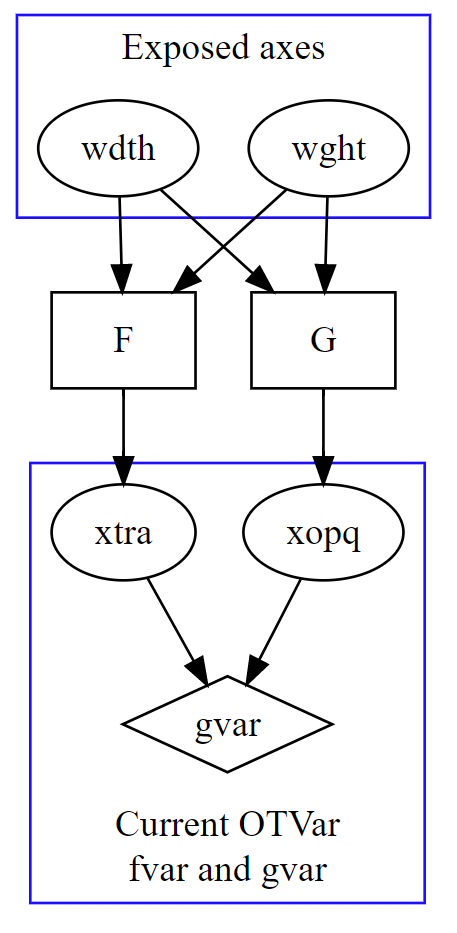#### Howdy, Stranger!

It looks like you're new here. If you want to get involved, click one of these buttons!

# [OTVar] Dependent axes?

As saw the axes proposal from Type Network, it worth saying that, if the fvar can support dependent axes, it would help solving some complex situations (like building a composite font from two VFs with different avar, or providing controls of non-orthogonal concepts).

Given that axes in fvar are almost Lambdas, the concept of dependent axes are Let-bindings. More precisely, a dependent axis is a "variable quantity" on the space of exposed axes, and could be used by the rest parts of the font (like gvar). For example, a user may define a dependent axis "stem width" in a font with one exposed axis "weight":

>> stem-width = F(weight) << // where F is a segmentwise linear function within range [-1, 1], represented in delta-tuples

then in the rest parts of the font, they would think that the font is two-dimensional, with first axis being "Weight" and second being "Stem width".

If dependent axes are present then the entire avar table is needless (since we can add the "adjusted" axes as individual dependent axes, while the original axes are still usable).

• So far, we have ideas around virtual axes, synthetic axes, and now dependent axes.

What seems clear is that we need some mechanism by which manipulation of design by axes that affect individual parameters can be mapped to axes that affect the visual appearance of the design.
• So far, we have ideas around virtual axes, synthetic axes, and now dependent axes.

What seems clear is that we need some mechanism by which manipulation of design by axes that affect individual parameters can be mapped to axes that affect the visual appearance of the design.
The ideas are similar: the existing fvar are Lambdas, and the new thing are Let bindings.
• So far, we have ideas around virtual axes, synthetic axes, and now dependent axes.
What is actually different about these, though? They all seems like different names for the same thing, to me.
• edited December 2017
I propose a conceptual difference between virtual and synthetic axes (I can't claim to really understand @Belleve Invis dependent axis idea yet, because I'm not strong on the math side and don't know what Lambdas and Let bindings are).

I think of a virtual axis as a involving mapping one or more gvar axes to a gvar-less axis; whereas, the term synthetic axis could also refer to a gvar axis that is synthesised from other axes (so, if I've understood correctly, some of the Amstelvar axes are synthesised from the parametric axes, right?). I reckon there's probably implementational overlap between the two concepts, i.e. it would be possible to make a synthetic virtual axis (which perhaps describes Rob McKaughn's XVAR implementation idea).
• Perhaps ytlc is a virtual axis, in that it is a subset of the ytra axis (and in fact really there situation is the opposite: ytra is the sum of the other Y transparent axes) and opsz is a synthetic axis in that it's tracing a path through the design space created by the x and y opaque and transparent axes.
• I propose a conceptual difference between virtual and synthetic axes (I can't claim to really understand @Belleve Invis dependent axis idea yet, because I'm not strong on the math side and don't know what Lambdas and Let bindings are).

I think of a virtual axis as a involving mapping one or more gvar axes to a gvar-less axis; whereas, the term synthetic axis could also refer to a gvar axis that is synthesised from other axes (so, if I've understood correctly, some of the Amstelvar axes are synthesised from the parametric axes, right?). I reckon there's probably implementational overlap between the two concepts, i.e. it would be possible to make a synthetic virtual axis (which perhaps describes Rob McKaughn's XVAR implementation idea).
Dependent axes are the combination of both the concepts, and much more powerful: a dependent axis defines an equation that performs delta-tuple interpolations of "exposed" axes. So one dependent axis could depend on on or multiple exposed axes.
• edited January 2018
An image for explaining this idea:The current FVAR-GVAR combination is the blue box on the bottom. Dependent Axes is mostly adding two high layers on the top of it: the first is a set of axes, which are exposed to the end user; the second are a (or multiple) sets of functions, which connects the "exposed axes" and "internal axes".

 “All problems in computer science can be solved by another level of indirection.”
• This sounds like idea of taking the XVAR table proposal and applying it as an implementation for "virtual" ("synthetic"/"meta") axes.
• @Peter Constable

Maybe we can combine the ideas: let "XVAR" to include more "result axes"? Or making it lie before "fvar"?

The code below is my idea, represented in C++-like type definitions.

```class Table_AXES {
Uint16 majorVersion; // set to 1
Uint16 minorVersion; // set to 0
Uint16 numberOfFvarAxes;   // identical to the axisCount in FVAR
Uint16 numberOfDesignAxes;
Uint16 numberOfMappers;
Vector<numberOfDesignAxes, Offset32<AxisDef>> designAxes;
Vector<numberOfMappers, Offset32<Mapper<numberOfFvarAxes>>> mappers;
}

class AxisDef {
Tag axisTag;
Fixed minValue;
Fixed defaultValue;
Fixed maxValue;
Uint16 flags;
Uint16 axisNameID;
}

template <Uint16 nFVA>
class Mapper {
Uint16 formulaVersion; // set to 1
Tag layoutMode;        // 'hori' or 'vert' for now
Vector<nFVA, Formula<formulaVersion>> formulae;
}

template <Uint16 formulaVersion>
class Formula {}

template<>
class Formula<1> {
Fixed constantTerm;
Uint16 numberOfPolymorphicTerms;
Vector<numberOfPolymorphicTerms, Format1Term> terms;
}

class Format1Term {
Uint16 numberOfFactors;
Vector<numberOfFactors, Format1Factor> factors;
}

class Format1Factor {
Tag designAxisTag;
Fixed coefficient;
Fixed minValue;
Fixed peakValue;
Fixed maxValue;
}
```